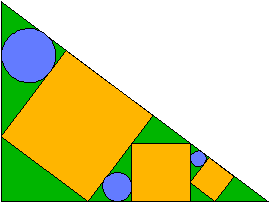# Three Circles in the Temple

Suppose the largest circle in the following figure has radius 7569 and the smallest has radius 100. What is the radius of the middle circle? The diagram below is followed by a description and the Mathematica code used to generate it.Source: This is a Japanese Temple problem. Hundreds of geometry problems are inscribed on wood and installed at temples in Japan. The May 1998 issue of Scientific American has an article on this subject; and a book was published in 1989 containing a large collection of such problems: "Japanese Temple Geometry Problems, San Gaku", by Hidetosi Fukagawa and Dan Pedoe (Charles Babbage Research Center, P. O. Box 272, St. Norbert Postal Station, Winnipeg, Canada R3V)

#### Description

Take a right angled triangle T with right angle at the origin and two legs lying along the positive x and y axes. Draw square1 having one side on the hypotenuse of T and its other two vertices lying one on each leg.

In the right triangle now appearing on the right side of the figure, inscribe square2 according to the same rules (with respect to its hypotenuse and legs). In the new right triangle that then appears at the far right, draw square3 using the same rules (with respect to its hypotenuse and legs). The resulting figure has 7 right triangles positioned as follows:

```1
4
5
2      3         6   7
```
where circle1 is the circle inscribed in right triangle 1, circle3 is the circle inscribed in right triangle 3, and circle5 is the circle inscribed in right triangle 5.

In words, let circle1 be inscribed in the right triangle at upper left. Let circle2 be inscribed in the small triangle whose sides are part of a side of square1, a side of square2, and the horizontal leg of T. And let circle3 be inscribed in the triangle whose sides are a side of square3, part of a side of square2, and part of the hypotenuse of T.

#### Mathematica Code for the Diagram

```Distance[u_, v_] := Norm[u - v]
Norm[p_] := Sqrt[p . p]

Module[{s = Perimeter[triangle]/2},
Sqrt[Times @@ (s - SideLengths[triangle])/s]]

Perimeter[poly_] := Plus @@ SideLengths[poly]

Incenter[triangle_] :=
SideLengths[triangle] . RotateRight[triangle]/
Perimeter[triangle]

SideLengths[poly_] :=
Apply[Distance, Partition[extend[poly], 2, 1], {1}]

extend[a_] := Flatten[{a, {First[a]}}, 1]
c1 = RGBColor[0, 0.7, 0];
c2 = RGBColor[1, 0.7, 0.];
c3 = RGBColor[0.4, 0.5, 1];
a = 4; b = 3;
c := Sqrt[a^2 + b^2];
y := a*b^2/(a*b + c^2);
s := b*r1;
r1 = (a - y*a/b)/c; y1 = y*r1; s2 = s*r1;
xx = a/b*y + (s - y1)*b/c;
y2 = y*(a - (xx + s2))/a;
s3 := y2*Sqrt[1 + (a/b)^2];

poly[p_, c_] :=
{{c, Polygon[p]}, Line[Append[p, First[p]]]}

cir[c_, r_, col_] := {{col, Disk[c, r]},
Circle[c, r]}

Show[Graphics[{poly[{{0, 0}, {a, 0}, {0, b}}, c1],
poly[{p1 = {0, y}, p2 = {a*y/b, 0},
p3 = {x = a*y/b + a/c*(b - y)*b/c, (x - a*y/b)*a/b},
p4 = p3 + p1 - p2}, c2],
cir[Incenter[tri = {p1, p4, {0, b}}],
poly[{p1 = {xx, 0}, p2 = {xx, s2},
p3 = p2 + {s2, 0}, p4 = p1 + {s2, 0}}, c2],
cir[Incenter[tri = {p1, p2, {a/b*y, 0}}],